## Diagonal Matrix

A diagonal matrix is a Matrix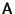of the form(1)

where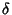is the Kronecker Delta,are constants, and there is no summation over indices. The general diagonal matrix is therefore Square and of the form(2)

Given a Matrix equation of the form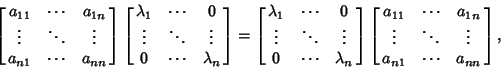(3)

multiply through to obtain(4)

Since in general,for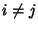, this can be true only if off-diagonal components vanish. Therefore, A must be diagonal.

Given a diagonal matrix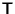,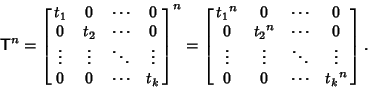(5)